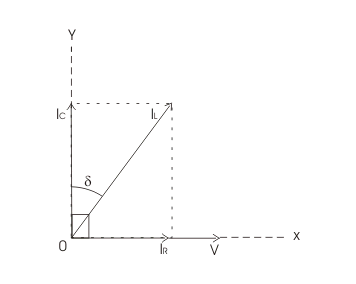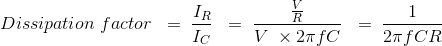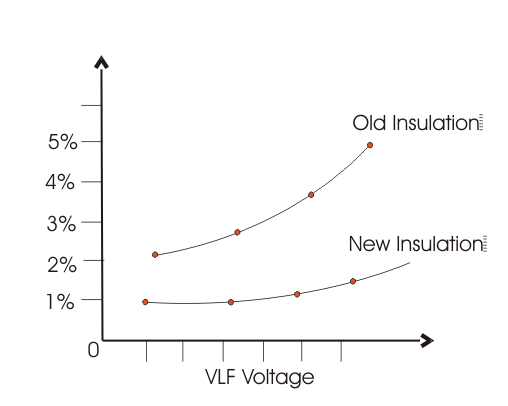# Tan Delta Test | Loss Angle Test | Dissipation Factor Test

## Principle of Tan Delta Test

A pure insulator when is connected across line and earth, it behaves as a capacitor. In an ideal insulator, as the insulating material which acts as dielectric too, is 100 % pure, the electric current passing through the insulator, only have capacitive component. There is no resistive component of the current, flowing from line to earth through insulator as in ideal insulating material, there is zero percent impurity.

In a pure capacitor, the capacitive electric current leads the applied voltage by 90o.
In practice, the insulator cannot be made 100% pure. Also due to the aging of insulators, the impurities like dirt and moisture enter into it. These impurities provide the conductive path to the current. Consequently, an electric leakage current flowing from line to earth through the insulator has a resistive component.

Hence, it is needless to say that, for good insulator, this resistive component of electric leakage current is quite low. In another way, the healthiness of an electrical insulator can be determined by the ratio of the resistive component to the capacitive component. For good insulator, this ratio would be quite low. This ratio is commonly known as tanδ or tan delta. Sometimes it is also referred to as dissipation factor.In the vector diagram above, the system voltage is drawn along the x-axis. Conductive electric current i.e. resistive component of leakage current, IR will also be along x-axis.
As the capacitive component of leakage electric current IC leads system voltage by 90o, it will be drawn along y-axis.
Now, total leakage electric current IL(Ic + IR) makes an angle δ (say) with y-axis.
Now, from the diagram above, it is cleared, the ratio, IR to IC is nothing but tanδ or tan delta.NB: This δ angle is known as loss angle.

## Method of Tan Delta Testing

The cable, winding, current transformer, potential transformer, transformer bushing, on which tan delta test or dissipation factor test to be conducted, is first isolated from the system. A very low-frequency test voltage is applied across the equipment whose insulation to be tested.

First, the normal voltage is applied. If the value of tan delta appears good enough, the applied voltage is raised to 1.5 to 2 times of normal voltage, of the equipment. The tan delta controller unit takes measurement of tan delta values. A loss angle analyzer is connected with tan delta measuring unit to compare the tan delta values at normal voltage and higher voltages and analyze the results.

During the test, it is essential to apply test voltage at a very low frequency.

### Reason of applying Very Low Frequency

If the frequency of the applied voltage is high, then capacitive reactance of the insulator becomes low, hence the capacitive component of electric current is high. The resistive component is nearly fixed; it depends upon applied voltage and conductivity of the insulator. At high frequency as capacitive current, is large, the amplitude of the vector sum of capacitive and resistive components of electric current becomes large too.

Therefore, required apparent power for tan delta test would become high enough which is not practical. So to keep the power requirement for this dissipation factor test, very low-frequency test voltage is required. The frequency range for tan delta test is generally from 0.1 to 0.01 Hz depending upon size and nature of insulation.

There is another reason for which it is essential to keep the input frequency of the test as low as possible.

As we know,That means, dissipation factor tanδ ∝ 1/f.
Hence, at low frequency, the tan delta number is higher, and the measurement becomes easier.

### How to Predict the Result of Tan Delta Testing

There are two ways to predict the condition of an insulation system during tan delta or dissipation factor test.

First, one is, comparing the results of previous tests to determine, the deterioration of the condition of insulation due to aging effect.

The second one is, determining the condition of insulation from the value of tanδ, directly. No requirement of comparing previous results of tan delta test.

If the insulation is perfect, the loss factor will be approximately the same for all range of test voltages. But if the insulation is not sufficient, the value of tan delta increases in the higher range of test voltage.From the graph, it is clear that the tan and delta number nonlinearly increases with increasing test very low-frequency voltage. The increasing tan&delta, means, high resistive electric current component, in the insulation. These results can be compared with the results of previously tested insulators, to take the proper decision whether the equipment would be replaced or not.

Want To Learn Faster? 🎓
Get electrical articles delivered to your inbox every week.
No credit card required—it’s 100% free.Electrical4U is dedicated to the teaching and sharing of all things related to electrical and electronics engineering.

### 6 thoughts on “Tan Delta Test | Loss Angle Test | Dissipation Factor Test”

1.Very good &easily understandable article. Thanks for sharing

•No worries Venkat – thank you for your kind words.

2.Very useful and easily understandable article.

•Thank you Amit – very happy to hear!

3.•